Application of fractional calculus in the dynamics of beams

Abstract

This paper deals with a viscoelastic beam obeying a fractional differentiation constitutive law. The governing equation is derived from the viscoelastic material model. The equation of motion is solved by using the method of multiple scales. Additionally, principal parametric resonances are investigated in detail. The stability boundaries are also analytically determined from the solvability condition. It is concluded that the order and the coefficient of the fractional derivative have significant effect on the natural frequency and the amplitude of vibrations.

1 Introduction

Many researchers have demonstrated the potential of viscoelastic materials to improve the dynamics of fractionally damped structures. Fractional derivatives are practically used in the field of engineering for describing viscoelastic features in structural dynamics . Namely, linear or non-linear vibrations of axially moving beams have been studied extensively by many researchers . Fractional derivatives are used in the simplest viscoelastic models for some standard linear solid. It can be seen that the vibrations of the continuum are modeled in the form of a partial differential equation system . These damping models involve ordinary integer differential operators that are relatively easy to manipulate . On the other hand, fractional derivatives have more advantages in comparison with classical integer-order models .

The partial differential equations of fractional order are increasingly used to model problems in the continuum and other areas of application. The field of fractional calculus is of importance in various disciplines such as science, engineering, and pure and applied mathematics . The numerical solution for the time fractional partial differential equations subject to the initial-boundary value is introduced by Podlubny . The finite difference method for a fractional partial differential equation is presented by Zhang . Galucio et al. developed a finite element formulation of the fractional derivative viscoelastic model . Chen et al. studied the transient responses of an axially accelerating viscoelastic string constituted by the fractional differentiation law . Applications of the method of multiple scales to partial differential systems arising in non-linear vibrations of continuous systems were considered by Boyacı and Pakdemirli . The method of multiple scales is one of the most common perturbation methods used to investigate approximate analytical solutions of dynamical systems. The dynamic response of the continuum is analyzed by using this method.

In this paper, longitudinal vibrations of the beam with external harmonic force are studied. The model developed is used to show the applicability of the fractional damped model and to find an approximate solution of the problem. The Riemann-Liouville fractional operator is emphasized among several definitions of a fractional operator [10, 11]. On the other hand, the approximate solution of the beam modeled by a fractional derivative is obtained and an application of the fractional damped model is also given. Additionally, the effects of a fractional damping term on a dynamical system are investigated. Finally, it is seen that the fractional derivative also has an effect on damping as a result of the previous studies in the literature.

2 The equation of motion

The problem of giving the longitudinal vibration of a harmonic external forced beam is given by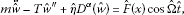(1)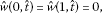(2)

where $w ˆ ( x ˆ , t ˆ )$ is the transverse displacements of the beam and ε is a small dimensionless parameter; m denotes the mass and $η ˆ$ is the damping coefficient; $F ˆ$ is the external excitation amplitude, $Ω ˆ$ is the external excitation frequencies, and $D α$ denotes the fractional derivative of order α. Here, also, the dot denotes partial differentiation with respect to time $t ˆ$, and prime denotes the derivative with respect to spatial $x ˆ$. On the other hand, it is assumed that the tension T is characterized as a small periodic perturbation $ε T 1 cosΩ$ on the steady-state tension $T 0$, i.e.,

$T= T 0 +ε T 1 cosΩ,$
(3)

where Ω is the frequency of a beam . Introducing the dimensionless parameters as

$w= w ˆ L ,x= x ˆ L ,t= t ˆ L T 0 ρ A ,$
(4)

we have the new dimensionless parameters

$a = T 1 T 0 , η ¯ = η ˆ L α − 2 T 0 α − 2 m α ( η ¯ = ε η ) , Ω 1 = Ω L m T 0 , Ω 2 = Ω ˆ L m T 0 , f ( x ) = L T 0 F ˆ ( x ) ,$
(5)

where ρ is density, A is the cross-sectional area, and L is the length of the beam. Thus, the equation in the non-dimensional form is presented as

$w ¨ −(1+εacos Ω 1 t) w ″ +εη D α w=f(x)cos Ω 2 t,$
(6)

where $η ¯$ equals εη. For simply supported beams, non-dimensional boundary conditions are

$w(0,t)=w(1,t)=0.$
(7)

3 The method of multiple scales

In this section, an approximate solution will be searched by using the method of multiple scales. This method is known as the direct-perturbation method which can be applied directly to the partial differential equation. In higher-order schemes and for finite mode truncations, the method yields better approximations to the real problem . Let us consider the expansion

$w(x,t;ε)= w 0 (x, T 0 , T 1 )+ε w 1 (x, T 0 , T 1 )+⋯,$
(8)

where $T 0 =t$ is the usual fast-time scale and $T 1 =εt$ is the slow-time scales. Now, the time derivatives are given by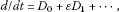(9)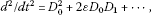(10)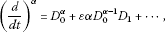(11)

where $D i =∂/∂ T i$. Here, $D + α e λ t = D 0 α e λ t = λ α e λ t$ can be used for calculating the fractional derivative of the exponential function, where $D + α , D + α − 1 , D + α − 2 ,…$ are the Riemann-Liouville fractional derivatives . Substituting Eqs. (8)-(11) into Eqs. (6) and (7) and separating into terms at each order of ε, we have the following: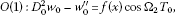(12)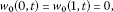(13)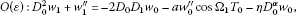(14)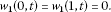(15)

At order one, the solution is obtained as

$w 0 ( x , t ) = [ A n ( T 1 ) e i ω n T 0 + A ¯ n ( T 1 ) e − i ω n T 0 ] X n ( x ) + [ e i Ω 2 T 0 + e − i Ω 2 T 0 ] Y ( x ) ,$
(16)

where $ω n$ represents the natural frequency, $A n$ and $A ¯ n$ are complex amplitudes and their conjugates, respectively. Now, substituting (16) into Eq. (12), we obtain the boundary value problems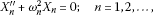(17)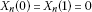(18)

and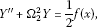(19)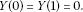(20)

Thus, the solutions of Eqs. (17) and (19) are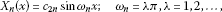(21)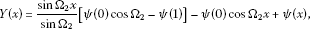(22)

where $ψ(x)$ is a particular solution for Eq. (19). Here, the particular solution of Eq. (19) changes with respect to the selection of the function $f(x)$. Let us substitute (16) into Eq. (14) for the solution of order ε, then

$D 0 2 w 1 + L 0 [ w 1 ] = [ − 2 i ω n D 1 A n e i ω n T 0 + 2 i ω n D 1 A ¯ n e − i ω n T 0 ] X n ( x ) + a 2 X n ″ [ A n ( e i ( ω n + Ω 1 ) T 0 + e i ( ω n − Ω 1 ) T 0 ) + A ¯ n ( e − i ( ω n − Ω 1 ) T 0 + e − i ( ω n + Ω 1 ) T 0 ) ] + a 2 Y ″ [ e i ( Ω 1 + Ω 2 ) T 0 + e − i ( Ω 1 + Ω 2 ) T 0 + e i ( Ω 1 − Ω 2 ) T 0 + e − i ( Ω 1 − Ω 2 ) T 0 ] − η X n [ ( i ω n ) α A n e i ω n T 0 + ( − i ω n ) α A ¯ n e − i ω n T 0 ] − η Y [ ( i Ω 2 ) α e i Ω 2 T 0 + ( − i Ω 2 ) α e − i Ω 2 T 0 ] .$
(23)

Thus, different cases arise depending on the numerical value of variation frequency. These cases will be treated in the following sections.

4 Case studies

In this section, we assume that one dominant mode of vibrations exists. As a result of the previous studies in the literature, it is seen that the results are the same in the finite mode analysis and in the infinite mode analysis [3, 14]. Therefore, we consider one dominant mode of vibration in this study.

4.1 $Ω 1$ close to 0, $Ω 2$ away from $ω n$ ($Ω 1 ≅0$, $Ω 2 ≠ ω n$)

For this case, we consider the case of the nearness of $Ω 1$ to zero is expressed as

$Ω 1 ≅ε σ n ,$
(24)

where $σ n$ is a detuning parameter. Then, Eq. (23) becomes

$D 0 2 w 1 − w 1 ″ = [ − 2 i ω n D 1 A n X n + a 2 A n X n ″ ( e i ε σ n T 0 + e − i ε σ n T 0 ) − η ( i ω n ) α A n X n ] e i ω n T 0 + cc + NST$
(25)

where cc and NST denote complex conjugates and non-secular terms, respectively. Thus, the solution of Eq. (25) is

$w 1 = φ n (x, T 1 ) e i ω n T 0 + φ ¯ n (x, T 1 ) e − i ω n T 0 +W(x, T 0 , T 1 ),$
(26)

where the first term is related to the secular terms and the second term is related to the non-secular terms. Now, substituting Eq. (26) into Eq. (25), we obtain the equation

$φ n ″ + ω n 2 φ n =2i ω n D 1 A n X n −a A n X n ″ cos σ n T 1 +η ( i ω n ) α A n X n$
(27)

with the boundary conditions

$φ n (0)=0, φ n (1)=0.$
(28)

Using the solvability condition , we then find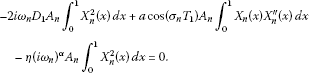(29)

Thus, by the normalization given as $∫ 0 1 X n 2 (x)dx=1$, then Eq. (29) turns into

$2i ω n D 1 A n + A n [ a cos ( σ n T 1 ) d 1 + ( i ω n ) α ] =0,$
(30)

where

$d 1 = ∫ 0 1 X n (x) X n ″ (x)dx.$
(31)

Then, the amplitude solution for the first order of the problem is as follows:

$A n ( T 1 ) = A 0 exp [ − η 2 ω n α − 1 T 1 sin ( π 2 α ) + i ( − a 2 ω n σ n sin ( σ n T 1 ) + η 2 ω n α − 1 cos ( π 2 α ) T 1 ) ]$
(32)

and the displacement is also obtained:

$w ( x , t ) ≅ A 0 exp ( − η 2 ω n α − 1 ε t sin ( π 2 α ) ) { exp [ − i a 2 ω n σ n sin ( σ n ε t ) ∫ 0 1 X n X n ″ d x + i η 2 ε t ω n α − 1 cos ( π 2 α ) + i ω n t ] + cc } sin ω n x + 2 cos Ω ¯ 2 t × [ sin Ω ¯ 2 x sin Ω ¯ 2 [ ψ ( 0 ) cos Ω ¯ 2 − ψ ( 1 ) ] − ψ ( 0 ) cos Ω ¯ 2 x + ψ ( x ) ] ,$
(33)

where $A 0$ is a constant (determined by enforcing initial conditions). Additionally, the supplementary natural frequency from the fractional derivative is also given by

$ω n a =− a 2 ω n σ n sin( σ n εt) ∫ 0 1 X n X n ″ dx+ η 2 εt ω n α − 1 cos ( π 2 α ) .$
(34)

As seen in Figure 1, the fractional derivative α-order has an effect on the displacement-time curves. In Figure 2 and Figure 3, the effect of the variation of the coefficient λ is observed for the different functions on displacement-time curves.

4.2 $Ω 1$ close to $2 ω n$, $Ω 2$ away from $ω n$ ($Ω 1 ≅2 ω n$, $Ω 2 ≠ ω n$)

If we consider the parametric resonance, then

$Ω 1 =2 ω n +ε σ n .$
(35)

Hence, the solvability condition requires that

$2i ω n D 1 A n − a 2 d 1 A ¯ n e i ε σ n T 1 +η ( i ω n ) α A n =0,$
(36)

where $d 1$ is given by (31). To perform the stability analysis, one introduces the transformation

$A n ( T 1 )= B n ( T 1 ) e i σ n T 1 / 2 ,$
(37)

where $B n$ can be written as

$B n ( T 1 )= ( b n R + i b n I ) e λ T 1 .$
(38)

Substituting Eq. (38) into Eq. (37) and also obtaining the result placed into Eq. (36) (and separating into real and imaginary parts), we get

$[ λ + η 2 ω n α − 1 sin ( π 2 α ) − d 1 4 ω n + ( η 2 ω n α − 1 cos ( π 2 α ) − σ n 2 ) − d 1 4 ω n − ( η 2 ω n α − 1 cos ( π 2 α ) − σ n 2 ) λ + η 2 ω n α − 1 sin ( π 2 α ) ] [ b n R b n I ] = [ 0 0 ] .$
(39)

For a non-trivial solution ($b n R ≠0$, $b n I ≠0$), the determinant of the coefficient matrix must be

$[ λ + η 2 ω n α − 1 sin ( π 2 α ) ] 2 − [ ( d 1 4 ω n ) 2 − ( η 2 ω n α − 1 cos ( π 2 α ) − σ n 2 ) 2 ] =0.$
(40)

Here, λ also must be zero for the steady-state condition. Thus, the stability boundaries are determined as follows:

$σ n =η ω n α − 1 cos ( π 2 α ) ± ( d 1 2 ω n ) 2 − ( η ω n α − 1 sin ( π 2 α ) ) 2 .$
(41)

Inserting $σ 1$ into Eq. (29), we obtain

$Ω 1 =2 ω n +ε [ η ω n α − 1 cos ( π 2 α ) ± ( d 1 2 ω n ) 2 − ( η ω n α − 1 sin ( π 2 α ) ) 2 ]$
(42)

for the external excitation frequency. Thus, the two different values of $Ω 1$ denote the stability boundaries for small ε. Additionally, it is seen that the stability boundaries depend not only on natural frequency but also on α.

The variation of an unstable region for different values of λ is observed in Figure 4. Since the rigidity of the system is increased by decreasing the value of λ, the unstable region reduces expeditiously for smaller values of λ.

The variation of an unstable region with some different values of α for $λ=5$ and $η=5$ is shown in Figure 5. Here, it is expected that the critical value of a becomes zero for $α=0$. This situation is clearly observed in Figure 5. On the other hand, the unstable region diminishes while α is increasing. Finally, the effect of the variation of α on the critical value of a is presented in Figure 6. Figure 7 shows that critical value a changes nonlinearly with the order of fractional derivative.

4.3 $Ω 1$ away from $2 ω n$ and 0, $Ω 2$ away from $ω n$ ($Ω 1 ≠2 ω n$, 0, $Ω 2 ≠ ω n$)

This case corresponds to the absence of any resonances. Then, Eq. (23) turns into

$D 0 2 w 1 + L 0 [ w 1 ]= [ − 2 i ω n D 1 A n X n − η ( i ω n ) α A n X n ] e i ω n T 0 +cc+NST,$
(43)

where cc is a complex conjugate and NST denotes non-secular terms. Substituting Eq. (26) into Eq. (43), we obtain the equation

$φ n ″ + ω n 2 φ n =2i ω n D 1 A n X n +η ( i ω n ) α A n X n$
(44)

with the boundary conditions

$φ n (0)= φ n (1)=0.$
(45)

Using the solvability condition , we find

$2i ω n D 1 A n ∫ 0 1 X n 2 (x)dx+η ( i ω n ) α A n ∫ 0 1 X n 2 (x)dx=0.$
(46)

By the normalization, then Eq. (46) becomes

$2i ω n D 1 A n + ( i ω n ) α d 1 A n =0.$
(47)

Thus, the displacement is obtained as follows:

$w ( x , t ) ≅ A 0 exp ( − η 2 ω n α − 1 sin ( π 2 α ) ε t ) × [ exp ( i η 2 ε t ω n α − 1 cos ( π 2 α ) + i ω n t ) + cc ] sin ω n x + 2 cos Ω ¯ 2 t [ sin Ω ¯ 2 x sin Ω ¯ 2 [ ψ ( 0 ) cos Ω ¯ 2 − ψ ( 1 ) ] − ψ ( 0 ) cos Ω ¯ 2 x + ψ ( x ) ] .$
(48)

On the other hand, the amplitude is

$A n ( T 1 )= A 0 exp ( [ i 2 ω n α − 1 η cos ( π 2 α ) − η 2 ω n α − 1 sin ( π 2 α ) ] T 1 ) .$
(49)

The displacement-time variation for different values of α is seen in Figure 8. Also, it is shown that the damping increases while the value of coefficient λ diminishes in Figure 9.

4.4 $Ω 1$ away from $2 ω n$ and $Ω 2$ close to $ω n$ ($Ω 1 ≠2 ω n ≠0$, $Ω 2 ≅ ω n$)

This case deals with the primary resonance $Ω 2 = ω n +ε σ n$ when the frequency of the transverse loading is approximately equal to the natural frequency. Then, the steady-state solutions of amplitude-phase modulation equations and their stability can be discussed. Using the polar form

$A n = 1 2 a n ( T 1 ) e i β n ( T 1 )$
(50)

and substituting Eq. (50) into the equation below,

$2i ω n D 1 A n +η ( i ω n ) α A n +η ( i ω n ) α d 2 e i σ n T 1 =0,$
(51)

where

$d 2 = ∫ 0 1 X n (x)Y(x)dx,$
(52)

we then obtain

$a n ′ +i a n β n ′ −iη ω n α − 1 [ cos ( π 2 α ) + i sin ( π 2 α ) ] ( 1 2 a n + d 2 e i ( σ n T 1 − β n ) ) =0,$
(53)

where $γ n = σ n T 1 − β n$. Separating the equation into real and imaginary parts and also substituting the equation

$a n ′ = γ n ′ =0$
(54)

into Eq. (53), we find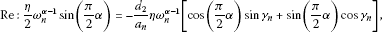(55)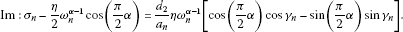(56)

By the same mathematical manipulation, the stability boundaries are calculated as follows:

$σ n = η 2 ω n α − 1 cos ( π 2 α ) ±η d 2 2 ω n 2 α − 2 a n 2 − ( 1 2 ω n α − 1 sin ( π 2 α ) ) 2 .$
(57)

4.5 Sum type of resonance ($Ω 1 + Ω 2 ≅ ω n +ε σ n$)

In this case, we consider the sum or difference of internal and external forced frequency since $Ω 1 ≠0$, $Ω 1 ≠2 ω n$, and $Ω 2 ≠ ω n$. Likewise, Eq. (23) is arranged once again; it is found that

$2i ω n D 1 A n + 1 2 d 3 e i σ n T 1 +η ( i ω n ) α A n =0,$
(58)

where

$d 3 =−a ∫ 0 1 X n (x)Y(x)dx.$
(59)

Substituting Eq. (50) into Eq. (58) and also separating the equation into real and imaginary parts, we get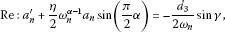(60)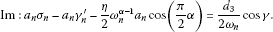(61)

Inserting Eq. (54) into Eqs. (60) and (61), then we have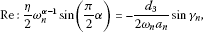(62)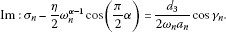(63)

Therefore, the stability boundaries are obtained as follows:

$σ n = η 2 ω n α − 1 cos ( π 2 α ) ± 1 2 d 3 2 ω n 2 a n 2 − η 2 ω n 2 α − 2 sin 2 ( π 2 α ) .$
(64)

5 Conclusion

In this study, the effects of the damping term modeled with a fractional derivative on the dynamic analysis of a beam having viscoelastic properties subject to the harmonic external force are investigated. The parametric or primary resonances in simple supported beams, the governing equation of which involves a fractional derivative, are also analyzed. It is concluded that the value of the natural frequency of the beam modeled with a fractional damper is greater than that of the beam modeled with a classical damper. The fractional derivative has no effect on the static behavior, but it has a significant impact on the dynamic behavior. Furthermore, it is seen that the unstable region in the resonance case diminishes when the order of the fractional derivative increases.

References

1. 1.

Rossikhin YA, Shitikova MV: Application of fractional calculus for dynamic problems of solid mechanics: novel trends and recent results. Appl. Mech. Rev. 2010., 63: Article ID 010801

2. 2.

Fung R-F, Huang J-S, Chen Y-C, Yao C-M: Nonlinear dynamic analysis of the viscoelastic string with a harmonically varying transport speed. Comput. Struct. 1998, 66(6):777–784. 10.1016/S0045-7949(98)00001-7

3. 3.

Pakdemirli M, Boyacı H: The direct-perturbation methods versus the discretization-perturbation method: linear systems. J. Sound Vib. 1997, 199(5):825–832. 10.1006/jsvi.1996.0643

4. 4.

Galucio AC, Deu J-F, Ohayon R: A fractional derivative viscoelastic model for hybrid active-passive damping treatments in time domain - application to sandwich beams. J. Intell. Mater. Syst. Struct. 2005, 16: 33–45. 10.1177/1045389X05046685

5. 5.

Podlubny I Mathematics in Science and Engineering 198. In Fractional Differential Equations. Academic Press, San Diego; 1999.

6. 6.

Agrawal OP: Formulation of Euler-Lagrange equations for fractional variational problems. J. Math. Anal. Appl. 2002, 272: 368–379. 10.1016/S0022-247X(02)00180-4

7. 7.

Zhang Y: A finite difference method for fractional partial differential equation. Appl. Math. Comput. 2009, 215: 524–529. 10.1016/j.amc.2009.05.018

8. 8.

Chen L-Q, Zhao W-J, Zu JW: Transient responses of an axially accelerating viscoelastic string constituted by a fractional differentiation law. J. Sound Vib. 2004, 278: 861–871. 10.1016/j.jsv.2003.10.012

9. 9.

Boyacı H, Pakdemirli M: A comparison of different versions of the method of multiple scales for partial differential equations. J. Sound Vib. 1997, 204(4):595–607. 10.1006/jsvi.1997.0951

10. 10.

Shimizu N, Zhang W: Fractional calculus approach to dynamic problems of viscoelastic materials. JSME Int. J. Ser. C Mech. Syst. Mach. Elem. Manuf. 1999, 42(4):825–837.

11. 11.

Rossikhin YA, Shitikova MV: Application of fractional calculus for analysis of nonlinear damped vibrations of suspension bridges. J. Eng. Mech. 1998, 124: 1029–1036. 10.1061/(ASCE)0733-9399(1998)124:9(1029)

12. 12.

Zhang L, Zu JW: Nonlinear vibration of parametrically excited moving belts, part I: dynamic response. J. Appl. Mech. 1999, 66(2):396–403. 10.1115/1.2791062

13. 13.

Öz HR, Pakdemirli M, Boyacı H: Non-linear vibrations and stability of an axially moving beam with time dependent velocity. Int. J. Non-Linear Mech. 2001, 36: 107–115. 10.1016/S0020-7462(99)00090-6

14. 14.

Pakdemirli M, Boyacı H: Comparison of direct-perturbation methods with discretization-perturbation methods for nonlinear vibrations. J. Sound Vib. 1995, 186: 837–845. 10.1006/jsvi.1995.0491

15. 15.

Nayfeh AH: Introduction to Perturbation Techniques. Wiley-Interscience, New York; 1981.

Author information

Correspondence to D Dönmez Demir.

Competing interests

The authors declare that they have no competing interests.

Authors’ contributions

All authors read and approved the final manuscript.

Authors’ original submitted files for images

Below are the links to the authors’ original submitted files for images.

Rights and permissions

Open Access This article is distributed under the terms of the Creative Commons Attribution 2.0 International License (https://creativecommons.org/licenses/by/2.0), which permits unrestricted use, distribution, and reproduction in any medium, provided the original work is properly cited.

Reprints and Permissions